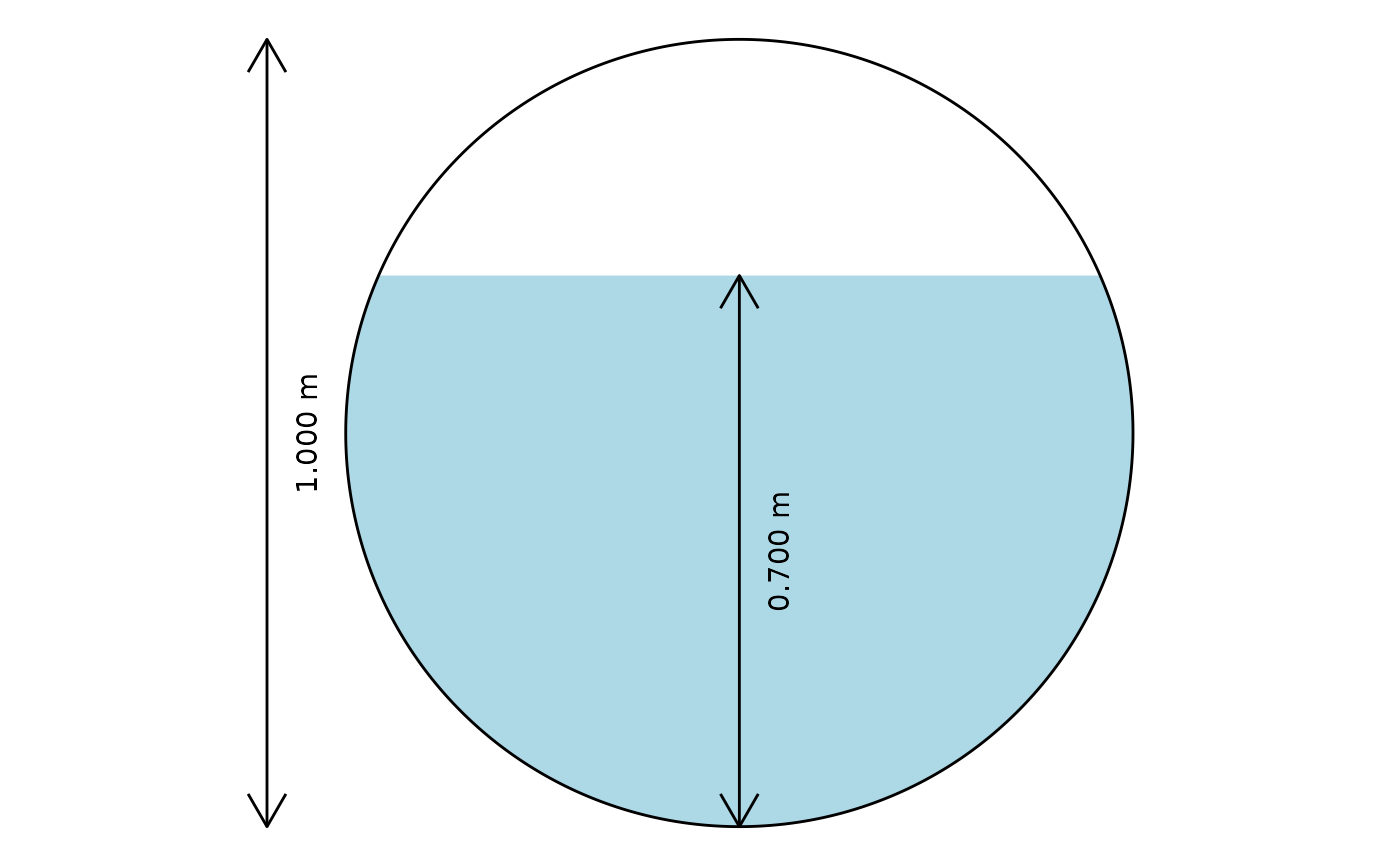This function plots a cross-section of a circular pipe, shaded as filled to the level indicated by the depth and diameter values passed to it.

## Usage

xc_circle(y = NULL, d = NULL, units = c("SI", "Eng"))

## Arguments

y

water depth [$$m$$ or $$ft$$]

d

pipe diameter [$$m$$ or $$ft$$]

units

character vector that contains the system of units [options are SI for International System of Units and Eng for English (US customary) units.

## Value

a cross-section diagram

Ed Maurer

## Examples


# Draw a cross-section with diameter 1.0 and depth 0.7
xc_circle(y = 0.7, d = 1.0, units = "SI")